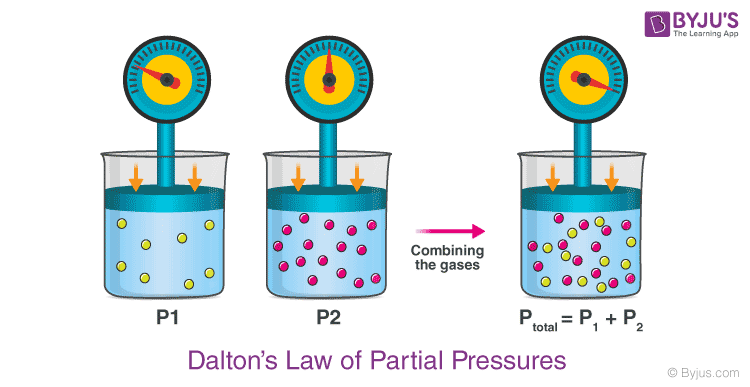Checkout JEE MAINS 2022 Question Paper Analysis : Checkout JEE MAINS 2022 Question Paper Analysis :

# Dalton's Law of Partial Pressure

## What is Dalton’s Law?

Dalton’s law of partial pressures is a gas law which states that the total pressure exerted by a mixture of gases is equal to the sum of the partial pressures exerted by each individual gas in the mixture.  For example, the total pressure exerted by a mixture of two gases A and B is equal to the sum of the individual partial pressures exerted by gas A and gas B (as illustrated below).## Dalton’s Law Formula

Dalton’s law of partial pressures can be mathematically expressed as follows:

Ptotal

$$\begin{array}{l}\sum_{i=1}^{n}p_{i}\end{array}$$
(or) Ptotal = P1 + P2 + P3 + …. + Pn

Where,

• Ptotal is the total pressure exerted by the mixture of gases
• P1, P2,…, Pn are the partial pressures of the gases 1, 2,…, ‘n’ in the mixture of ‘n’ gases

### Expressing Partial Pressures in Terms of Mole Fraction

The mole fraction of a specific gas in a mixture of gases is equal to the ratio of the partial pressure of that gas to the total pressure exerted by the gaseous mixture. This mole fraction can also be used to calculate the total number of moles of a constituent gas when the total number of moles in the mixture is known. Furthermore, the volume occupied by a specific gas in a mixture can also be calculated with this mole fraction with the help of the equation provided below.

$$\begin{array}{l}X_i = \frac{P_i}{P_{total}} = \frac{V_i}{V_{total}} = \frac{n_i}{n_{total}}\end{array}$$

Where Xi is the mole fraction of a gas ‘i’ in a mixture of ‘n’ gases, ‘n’ denotes the number of moles, ‘P’ denotes pressure, and ‘V’ denotes volume.

## Recommended Videos

#### Applying Concepts – Dalton’s law of partial pressure#### States of matter – Dalton law of partial pressure## Solved Examples on Dalton’s Law of Partial Pressure

### Example 1

A mixture of hydrogen gas and oxygen gas exerts a total pressure of 1.5 atm on the walls of its container. If the partial pressure of hydrogen is 1 atm, find the mole fraction of oxygen in the mixture.

Given, Phydrogen = 1 atm, Ptotal = 1.5 atm

Applying Dalton’s law formula, Ptotal = Phydrogen + Poxygen

Therefore, Poxygen = 0.5 atm

Now, the mole fraction of oxygen, Xoxygen = (Poxygen/Ptotal) = 0.5/1.5 = 0.33

Therefore, the mole fraction of oxygen in the mixture is 0.33

### Example 2

At a temperature of 300K, 30 litres of gas A kept under pressure of 1 atm and 15 litres of gas B kept under pressure of 2 atm is transferred into an empty 10L container. Calculate the total pressure inside the container and the partial pressures of gas A and gas B (Assume that A and B are ideal gases).

From the ideal gas equation, PV = nRT

Number of moles of gas A = (30L*1atm)/(0.08206 atm.L.mol-1.K-1 * 300K) = 1.22 mol

Number of moles of gas B = (15*2atm)/(0.08206 atm.L.mol-1.K-1 * 300K) = 1.22 mol

Total number of moles in the gaseous mixture = 2.44 mol

Total pressure inside the 10L container = Ptot = nRT/V

Ptot = (2.44mol*0.08206 atm.L.mol-1.K-1*300K)/10L = 6.006 atm

Therefore, the total pressure inside the 10 litre container is 6.006 atm

Mole fraction of gas A = mole fraction of gas B = (1.22 mol/2.44 mol) = 0.5

Therefore, partial pressure of gas A = Therefore, partial pressure of gas B = 0.5*6.006 = 3.003 atm

Thus, the partial pressures of gases A and B in the 10L container are both equal to 3.003 atm.